# Dynamic model

Our dynamic modeling is intended to present you the trend of concentration of various enzyme, protein or inducer involved in our genetic circuit. Since it would vividly show all the concentration of substance concerned relating to time .Together with data measured will it provide us the opportunity to predict the degradation velocity of 3pba.

The whole design is shown as follow: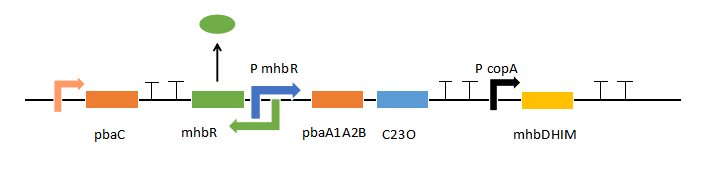It involve 3 gene cluster,pbaC pbaA1A2B,mhbRDHIM,C23O,which can express 6 kinds of enzymes and a TF and two promoters which need regulators. In order to make the system more easier to analyze we regard the electron transfer particle PbaC together with the enzyme complex PbaA1A2B as E1,and regard enzymes MhbD, MhbH, MhbI, MhbM as E2,as well as C23O as E3.

The equation of these enzymes,can be described as follow: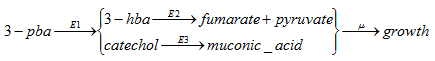In later part, E1,E2 and E3 will represent these enzymes in our model.

When it comes to the regulators,the promoter P mhbR can be regulated by MhbR and 3-hba while the promoter P lac can be regulated by lacI and IPTG.

So the whole dynamic modeling consist of two parts: 3-hba inducing and IPTG inducing.

## Part one-the 3-hba inducing

1.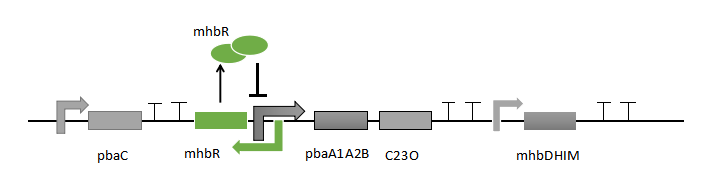2.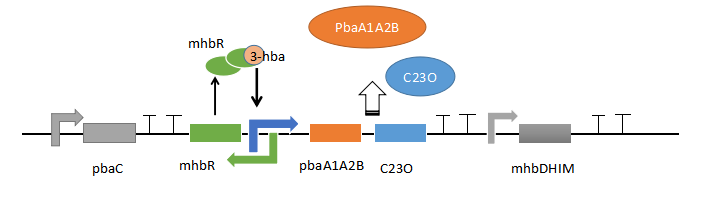Fig(1). A. When there is non-inducer mhbR repress the promoter. B. When there is 3-pba,they can be degraded into 3-hba,subsequently disinhibited the promoter.

mhbR is a Lys-R type transcription factor[] which will enable the transcription of the downstream gene cluster when binding 3-hba and will inhibit the process if MhbR itself binds mhbR the other way round.Although both two kinds of mhbR complex can bind the promoter,but the DNA will form different shape. Shape under the combination with mhbR_3-hba complex make transcription easier while the other shape will severely lower gene expression. Note that,when mhbR_3-hba complex bind the DNA,the expression of itself will be inhibited.

When there is no 3-pba outside the cell,genes pbaA1A2B and C23O will express a few enzyme,we described the process as follow:

nu_(mhbR_T)=a-k_(de)*mhbR_T

nu_(E1)=b_1-k_(de)*E_1

nu_(E3)=b_3-k_(de)*E_3

(a,b_1,b_3 represent the expression without 3-hba;k_(de) represent the degradation of enzymes.)

When there are 3-pba outside,they will enter into the cell in simple diffusion way,and we describe the process as follow :

nu_(3pba"_into")=-s*(pba"_outside"-pba"_inside")

nu_(pba"_inside")=s*(pba"_outside"-pba"_inside")-r_(E1);

r_(E1)=(m_1*E_1*pba"_inside"//K_(e1)^F-m_2*E_1*hba//E_(e1)^B)/(1+pba"_inside"//K_(e1)^F+hba//K_(e1)^B)

(define s as the osmotic coefficient, r_(Ei)as the enzyme-catalyzed reaction rate.We assume that the enzyme-catalyzed reaction can be modeled as Michaelis–Menten type kinetics, and is reversible);

Under the effect of PbaA1A2B complex,3-pba will be oxidized into 3-hba and catechol.3-pba's accumulation will activate the P mhbR.We describe the process as follow:

nu_(3-hba)=r_(E1)-r_(E2)

r_(E1)=(m1*E_1*pba"_inside"//K_(e1)^F-m_2*E_1*pba//K_(e1)^B)/(1+pba"_inside"//K_(e1)^F+hba//K_(e1)^B)

r_(E2)=(m_2*E_2*hba)/(K_(e2)+hba)

nu_(hba"_mhbR")="kon"*[mhbR"_free"]*[hba]-"koff"*[hba"_mhbR"]

nu_(MhbR_T)=a*(1-P_(mhbR"_promoter"))-k_(de)*mhbR"_free"-k_(de)*[hba"_mhbR"]

nu_(E_i)=b_(11)*P_(mhbR"_promoter")-k_(de)*E_1

MhbR_T=MhbR_("free")+hba"_MhbR"

Define P_(mhbR"_promoter") as the probability that the 3-hba_MhbR bind the promoter. 3-hba_MhbR complex and MhbR dimer compete to bind the promoter.

DNA_T=DNA_("free")+DNA"_MhbR"+DNA"_3-hba_MhbR"...(1)

nu_(DNA"_MhbR")=k_(on)DNA_("free")*MhbR_("free")-k_("off")DNA"_MhbR"...(2)

nu_(DNA"_3-hba_MhbR")=k_("on")DNA_("free")*"3-hba_MhbR"-k_("off")DNA"_3-hba_MhbR"...(3)

When steady, the nu_(DNA"_MhbR")=nu_("DNA_3-hba_MhbR")=0, then we can get:

[DNA"_3-hba_MhbR"]=(k_"on"*DNA_("free")*[3-hba"_MhbR"])/k_("off")...(4)

[DNA"_MhbR"]=(k_("on")*DNA_("free")*MhbR_("free"))/k_("off")...(5)

Only if the 3-hba band with DNA,can the prompter be activated. We define:

P_("mhbR_promoter")=([DNA"_3-hba_MhbR"])/(DNA_T)...(6)

Combine the function (1),(4),(5),(6),we can get:

P_("mhbR_promoter")=([hba"_mhbR"]*K_(mhbR"_p"))/(K_(mhbR"_p")*K_(mhbR"_hba_p")+[hba"_mhbR"]*K_("mhbR_p")+mhbR_("free")*K_("mhbR_hba_p"))

This part is the same as the feedforward loop,in which the product 3-hba of 3-pba 1,2-dioxygenase can combine with MhbR and help to disinhibition. So when 3-hba accumulate in the cell,the promoter becomes more active. It is a feedforward loop.

But 3-hba won't be allowed to accumulate all the way, then IPTG will be inputted and PlacI promoter disinhibited. With the expression the gene cluster mhbDHIM,3-hba will get degradation.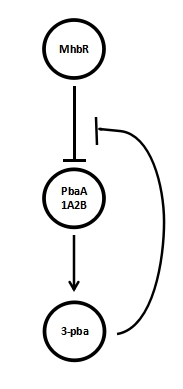Fig(2).The feedforeard loop

Variables Description Unit
nu_(mhbR) MhbR concentration changing rate mu"mol"*L^(-1)*"min"
nu_(Ei) nu_(Ei) concentration changing rate mu"mol"*L^(-1)*"min"
nu_(3pba"_into") 3-pba enter into the cell rate mu"mol"*L^(-1)*"min"
nu_(pba"_inside") 3-hba concentration changing rate mu"mol"*L^(-1)*"min"
nu_(hba"_mhbR") The complex concentration changing rate mu"mol"*L^(-1)*"min"
nu_(DNA"_mhbR") DNA and MhbR complex concentration changing rate mu"mol"*L^(-1)*"min"
nu_(DNA"_3hba_MhbR") DNA and 3-hba_MhbR complex concentration changing rate mu"mol"*L^(-1)*"min"
r_(Ei) enzyme-catalyzed (E1 E2 E3) reaction rate mu"mol"*L^(-1)*"min"
MhbR_T The whole concentration of MhbR mu"mol"*L^(-1)
MhbR_("free") The concentration of MhbR(don't combine with 3-hba) mu"mol"*L^(-1)

## Part two-- IPTG inducing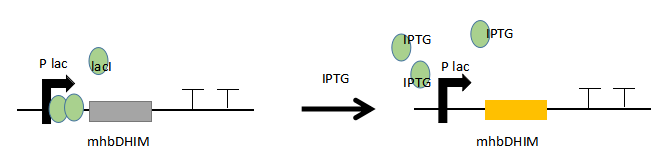Fig(3).IPTG can dis inhibited the Plac.

We'll input IPTG after adding 3-pba.IPTG will disinhibted the Plac promoter. And the gene cluster express the enzymes which enable 3-hba degraded into Fum and Pyr. The process can be described as follow.

When there is none IPTG:

nu_(lacI"_T")=a-k_(de)*lacI"_T"

nu_(E2)=(s*r_1)/(1+((lacI"_T")/K_(m1)^n)-k_(de)*lacI"_T"

lacI"_free"=lac"_T"

nu_(lacI"_T")=a-k_(de)*lacI"_free"-k_(de)*lac"_IPTG"

nu_(lacI"_IPTG")=k_("on")*lacI"_free"*IPTG-k_("off")*lacI"_IPTG"

nu_(E2)=alpha/(1+((lacI"_free")/K_(m1))^n)*E2

lacI"_free"=lacI"_T"-lacI"_IPTG"

Then 3-hba will be degraded by E2.

## Part three--Robustness

It's noted that once IPTG was added,they won't be consumed,as cell can't utilize them. We wondered if they were added too early to accumulate enough 3-pba which can help to product E1 and E3,does the system work still well?

We made a ideal simulation to find if our worries are necessary.fig(4).

A. B. C.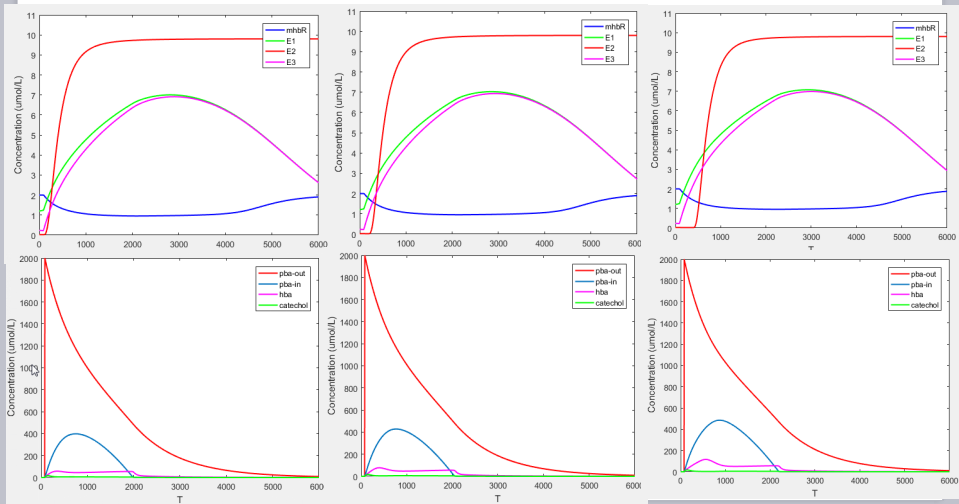D. E. F.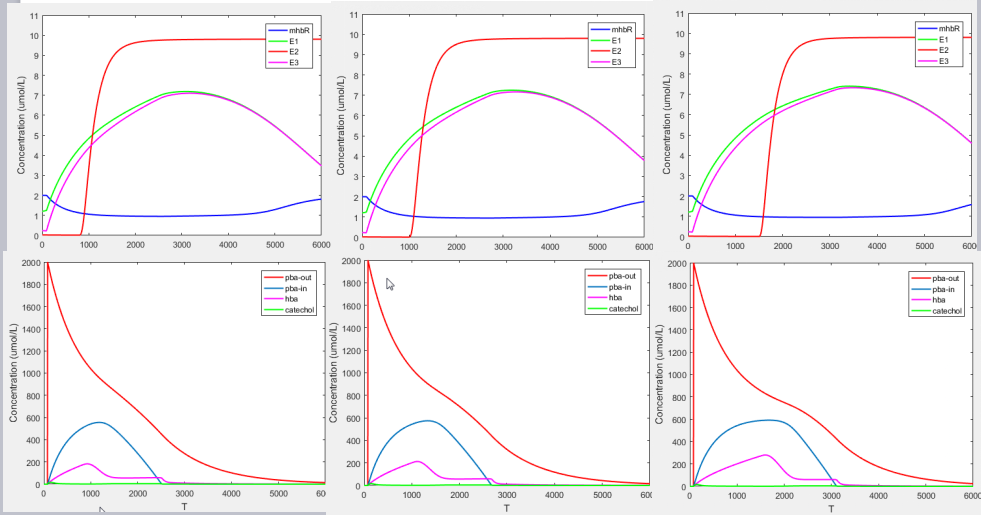Fig(4). The unit of time is minute. 3-pbe was added at 80 minute. From A to B,IPTG was added at different time.A(150min),B(250min),C(400min),D(800min),E(1000min),F(1500min).

These figures show that the 3-pba degrade rate fluctuate within a small range. When the enzymes degradation rate is slow, the system shows robustness.